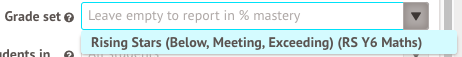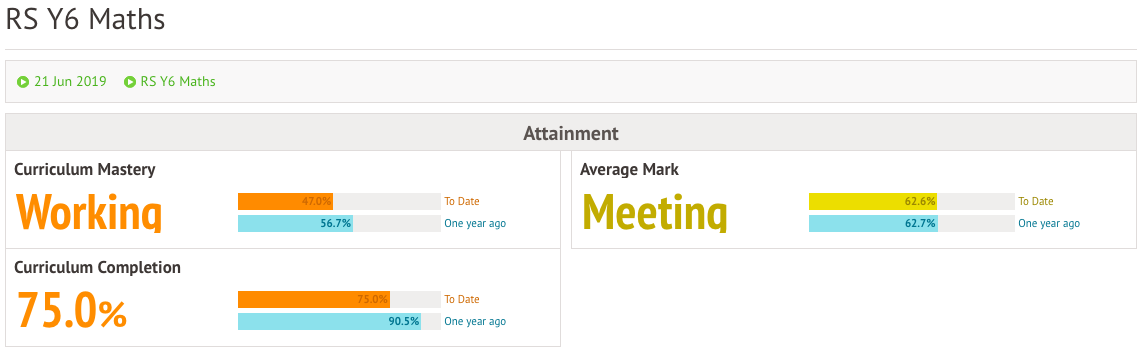# The Curriculum Analysis Overview

Go to Students > Assessments > Formative Tracking > Analysis.

This Overview page provides a snapshot of where the selection of students are in their mastery of the selected curriculum.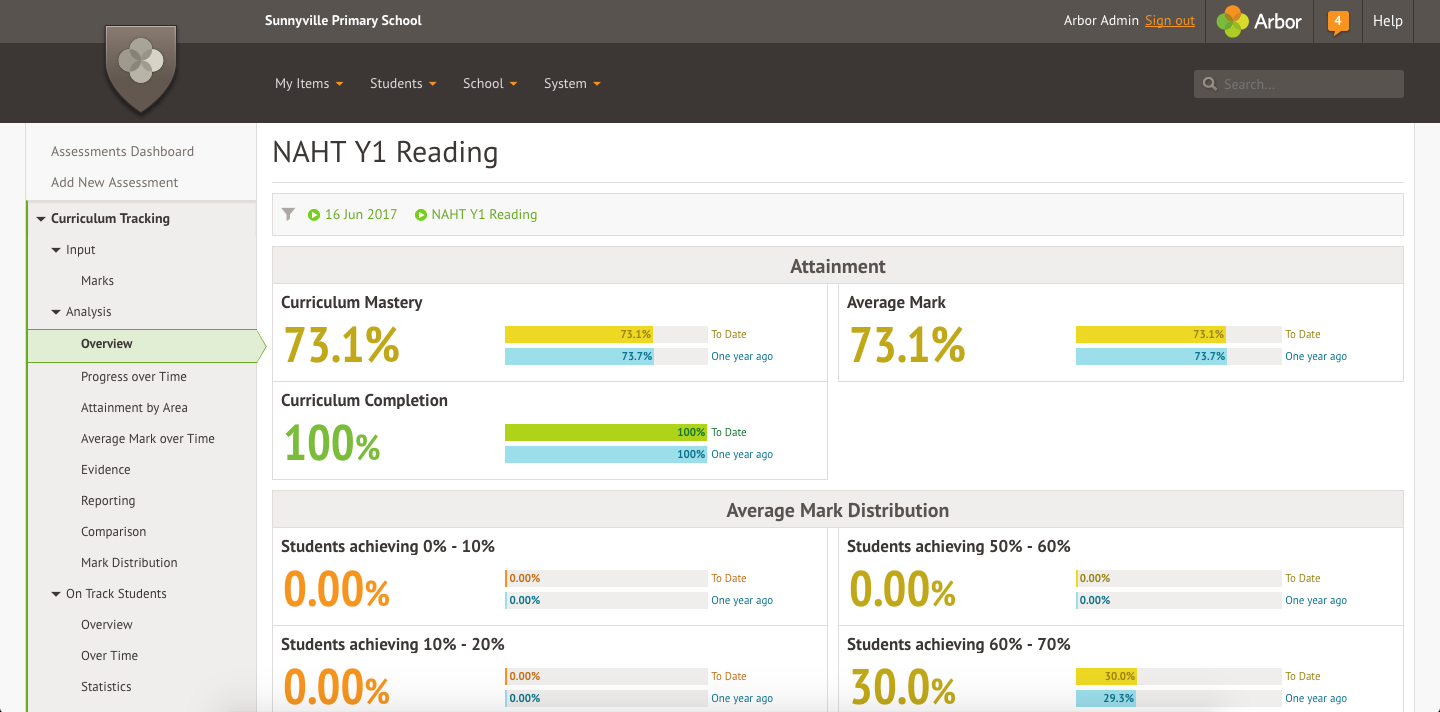Using the filters box have the option to choose to view the data for different curriculums, modules, students and more.

You can also view by different grade sets if you are using more than one in school.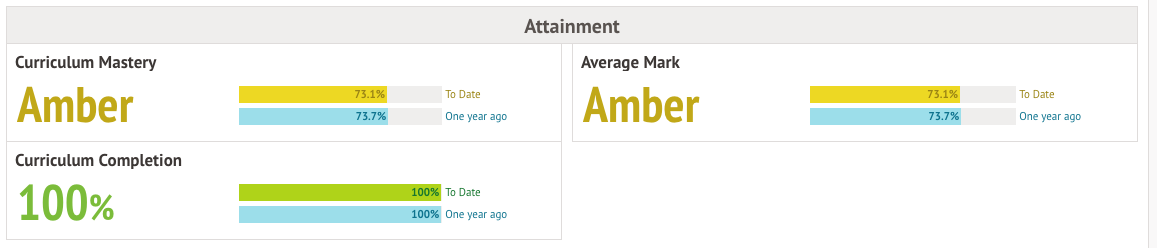## What is Curriculum Mastery?

### Curriculum Mastery

Curriculum Mastery refers to the average mark a student has out of the entire curriculum they are being assessed against. If a student was being assessed on a curriculum with 10 statements and only had 5 statements marked, the average comes from those 5 statements/10. To calculate the Curriculum Mastery, find the percentage completed and multiply that by the average mark.

The Curriculum Completion in the example below is 75%. This means that if there are 100 curriculum statements, 75 of them have been marked.

### Average Mark

The Average Mark is the average mark a student has ONLY on the curriculum statements that have been assessed. (If only 5 statements out of 10 have been marked then it is the overall average of the 5 marked statements.)

In the example below, the average mark is 62.6%. This means that if you average the mark of those 75 marked assessments, the average mark is 62.6%.

### Curriculum Completion

The Curriculum Completion is the % of the curriculum which has a mark against it. It is calculated by dividing the total number of statements assessed by the total number of possible statements.

To calculate the Curriculum Mastery for the example below, find 75% of the average mark. This calculation is 0.75 x 62.6 = 46.95, which rounds up to 47%. You can see this percentage under the Curriculum Mastery heading.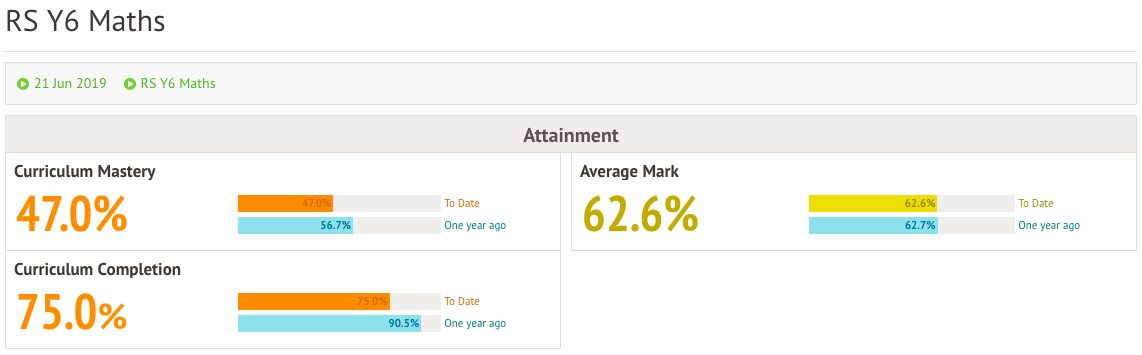## How is the percentage for Average Mark calculated?

Each mark given in the Curriculum Assessment marksheet is given a weighting between 0% and 100%. For example, you may have these options:

• Working towards = 33%
• Working at = 67%
• Working at greater depth = 100%

When finding the average mark, Arbor will round up or down to the nearest judgement. Here's some example average scores, and the overall judgement they correspond to.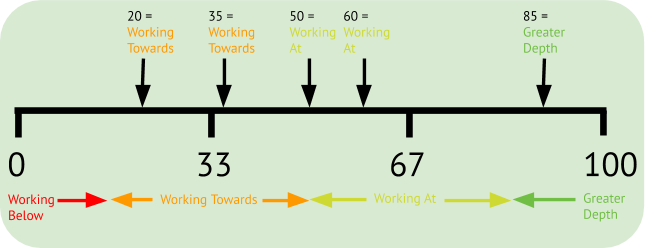### Top Tip

You can swap between viewing your statistics in percentages and the grades you have defined in the filters. Just click the box at the top of the page and select your Grade Set.